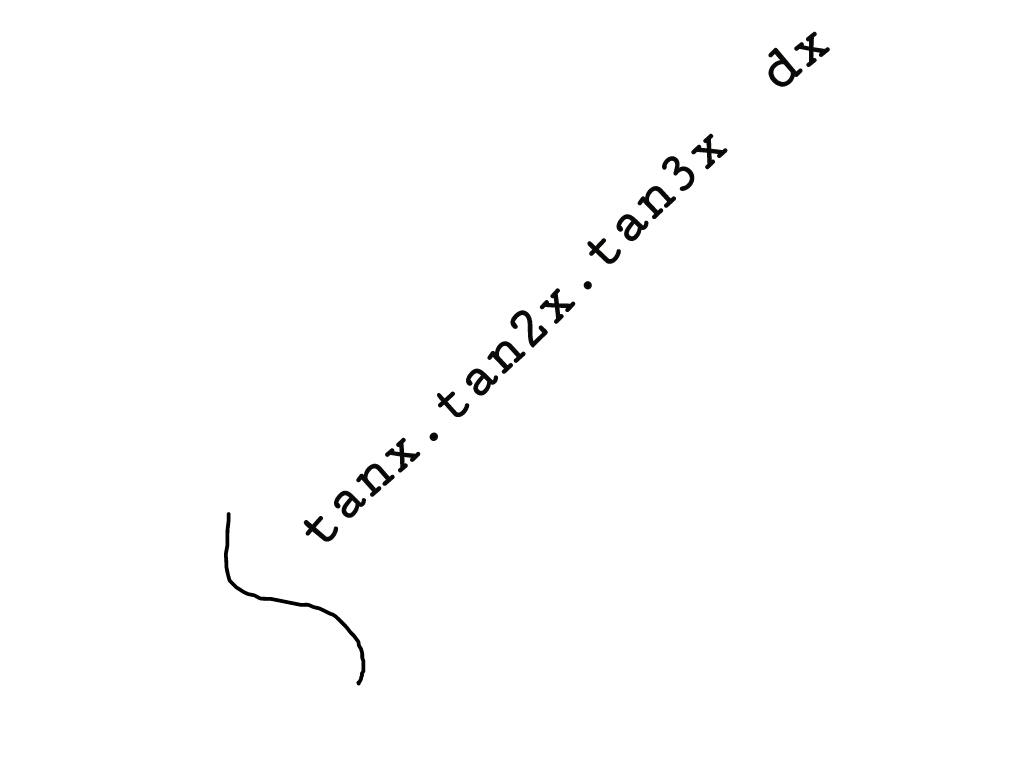# Integration of tanx tna2x tan3x dx please sir , give the answer this question7 years ago

(tanxtan2xtan3x)dx=ln(|sec(3x)|)3ln(|sec(2x)|)2ln(|secx|)+C

#### Explanation:

We can rewrite tanxtan2xtan3x in a way that is much easier to integrate. First, find out how else to write tan3x using the tangent angle addition formula.

tan(A+B)=tanA+tanB1tanAtanB

So, we see that:

tan3x=tan(x+2x)=tanx+tan2x1tanxtan2x

Cross-multiplying:

(1tanxtan2x)tan3x=tanx+tan2x

Distributing

tan3xtanxtan2xtan3x=tanx+tan2x

Solving for tanxtan2xtan3x:

tanxtan2xtan3x=tan3xtan2xtanx

Thus:

(tanxtan2xtan3x)dx=(tan3xtan2xtanx)dx

Splitting this apart:

=tan3xdxtan2xdxtanxdx

Note that tanxdx=ln(|secx|)+C. The first two integrals will require using substitution: let u=3xdu=3dx and v=2xdv=2dx.